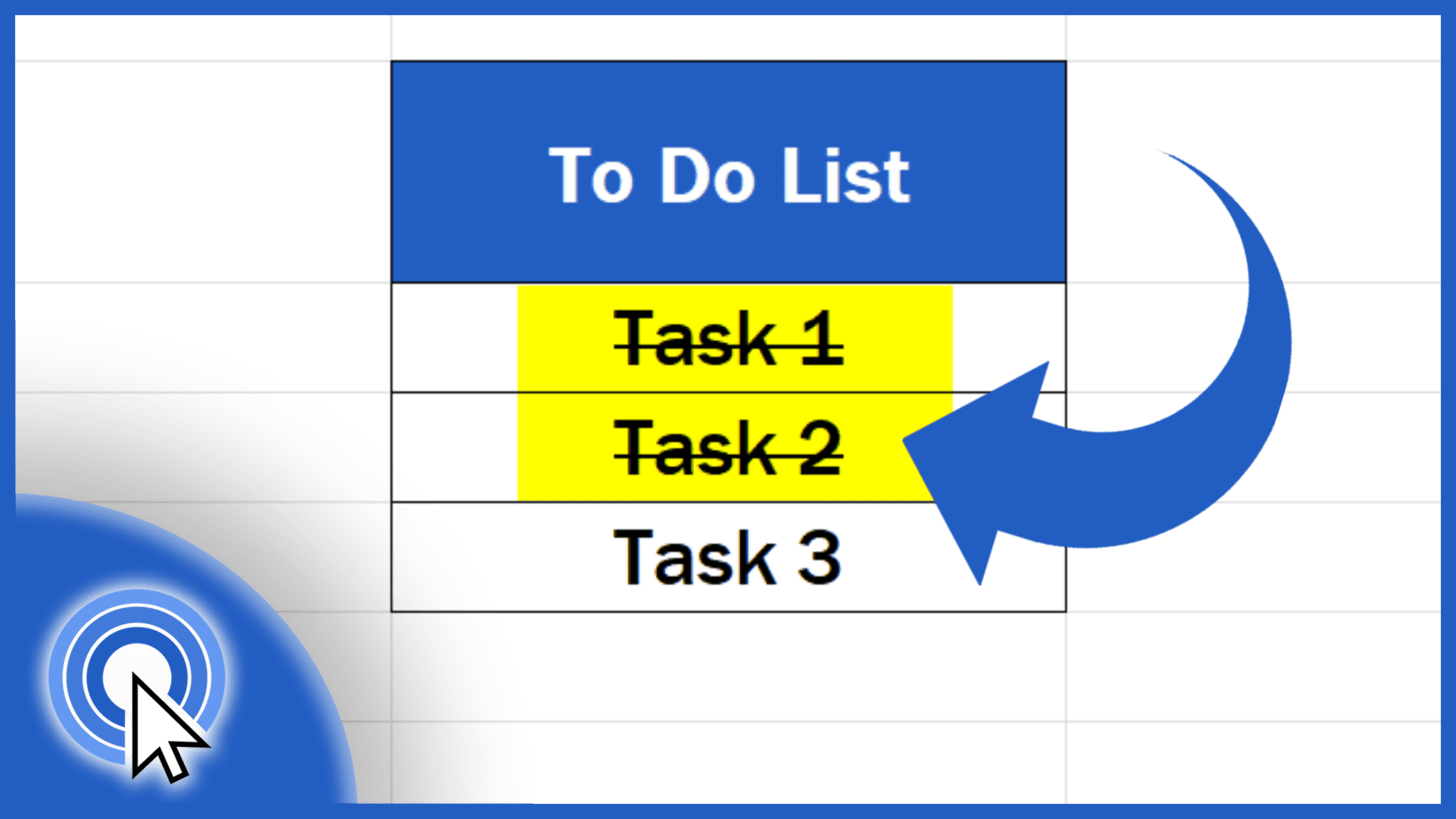# Category: Uncategorized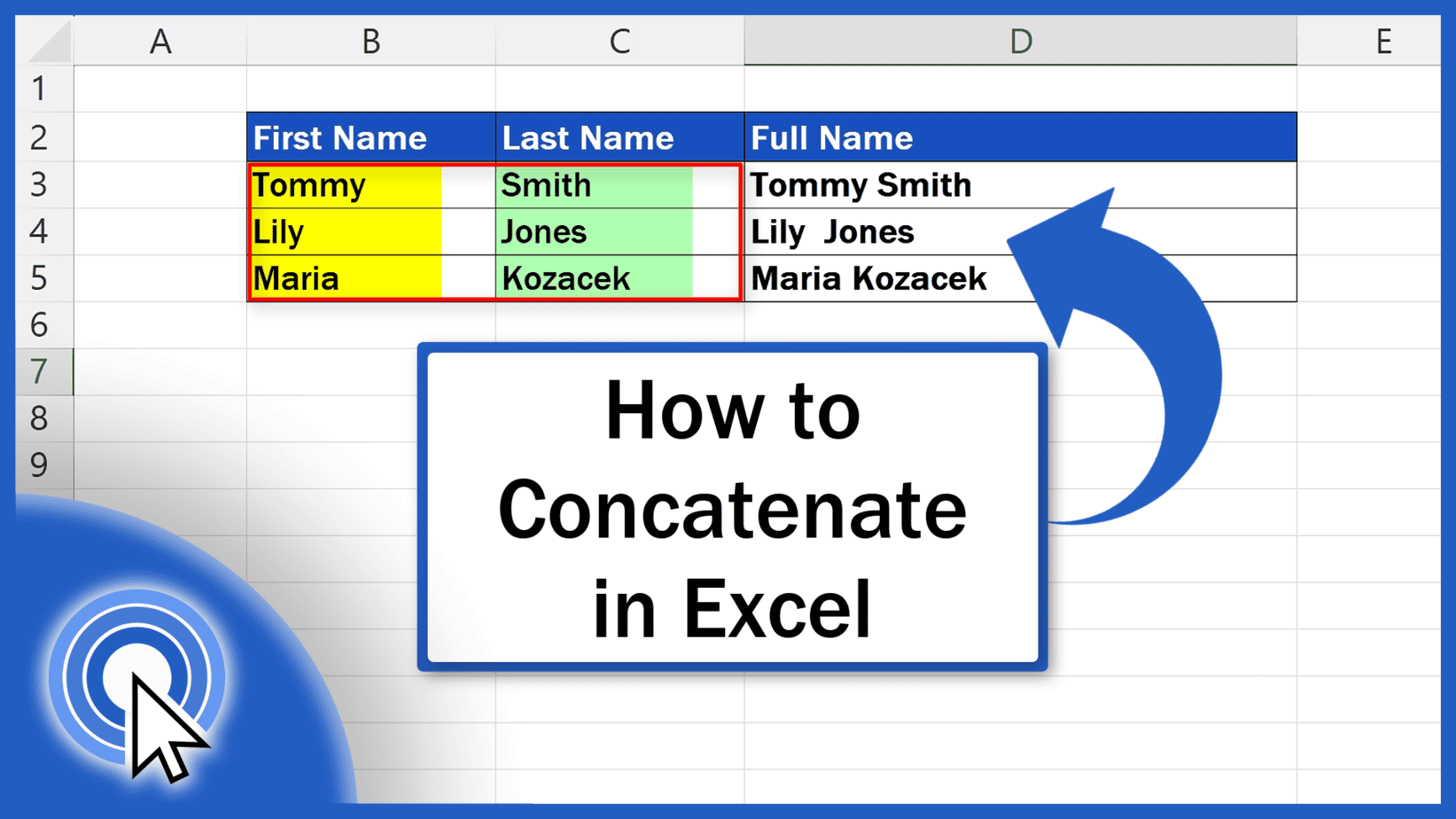## How to Concatenate in Excel (Quick and Easy)

In this tutorial we’re going to talk about how to concatenate in Excel. Thanks to this function, you’ll be able to link the contents of several different cells.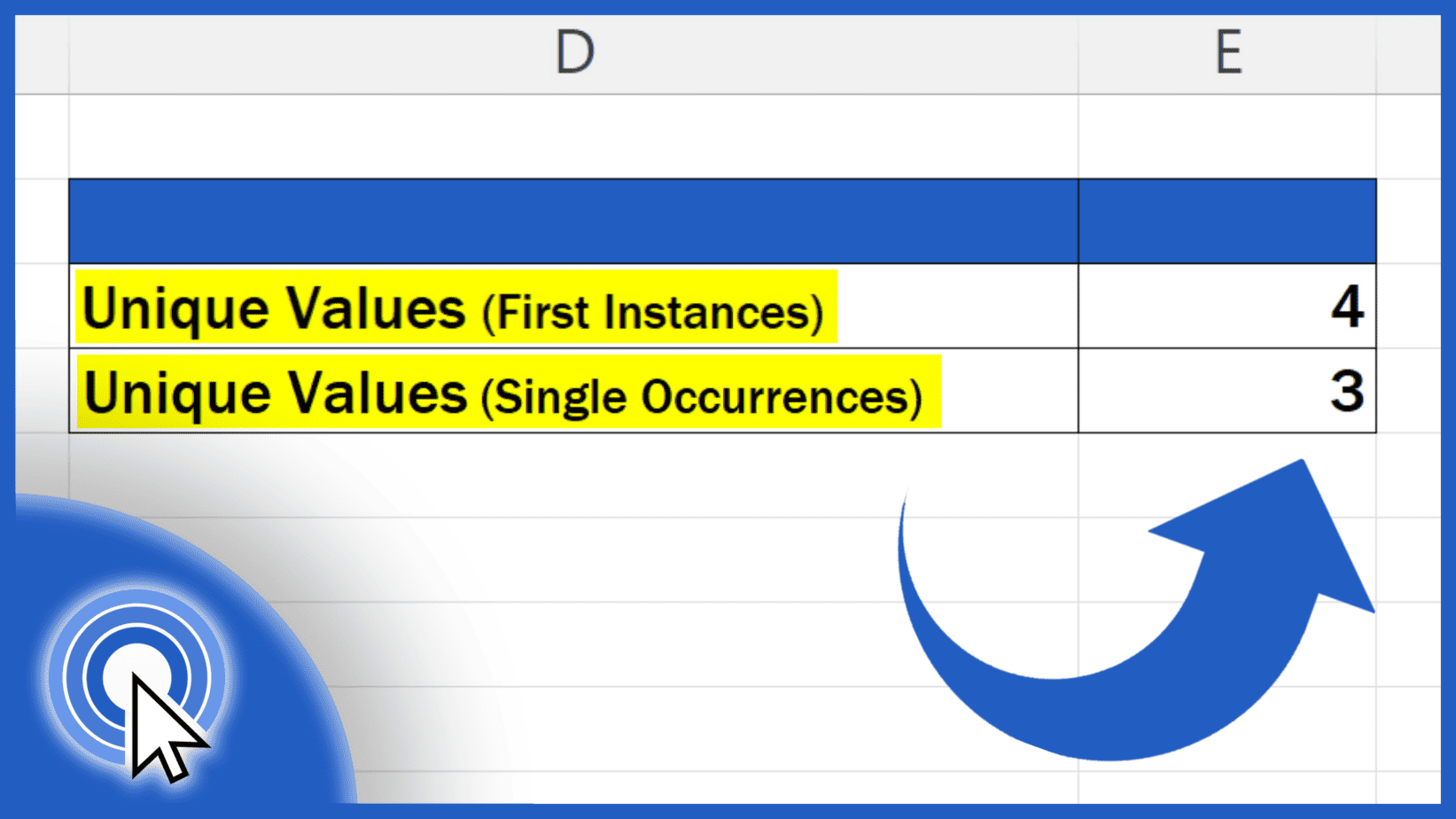## How to Count Unique Values in Excel (Quick and Easy)

Today we’re going to have a look at how to count unique values in Excel. We’ll be talking about two different senses of the word ‘unique’, hence two different ways to count unique values, both quick and easy.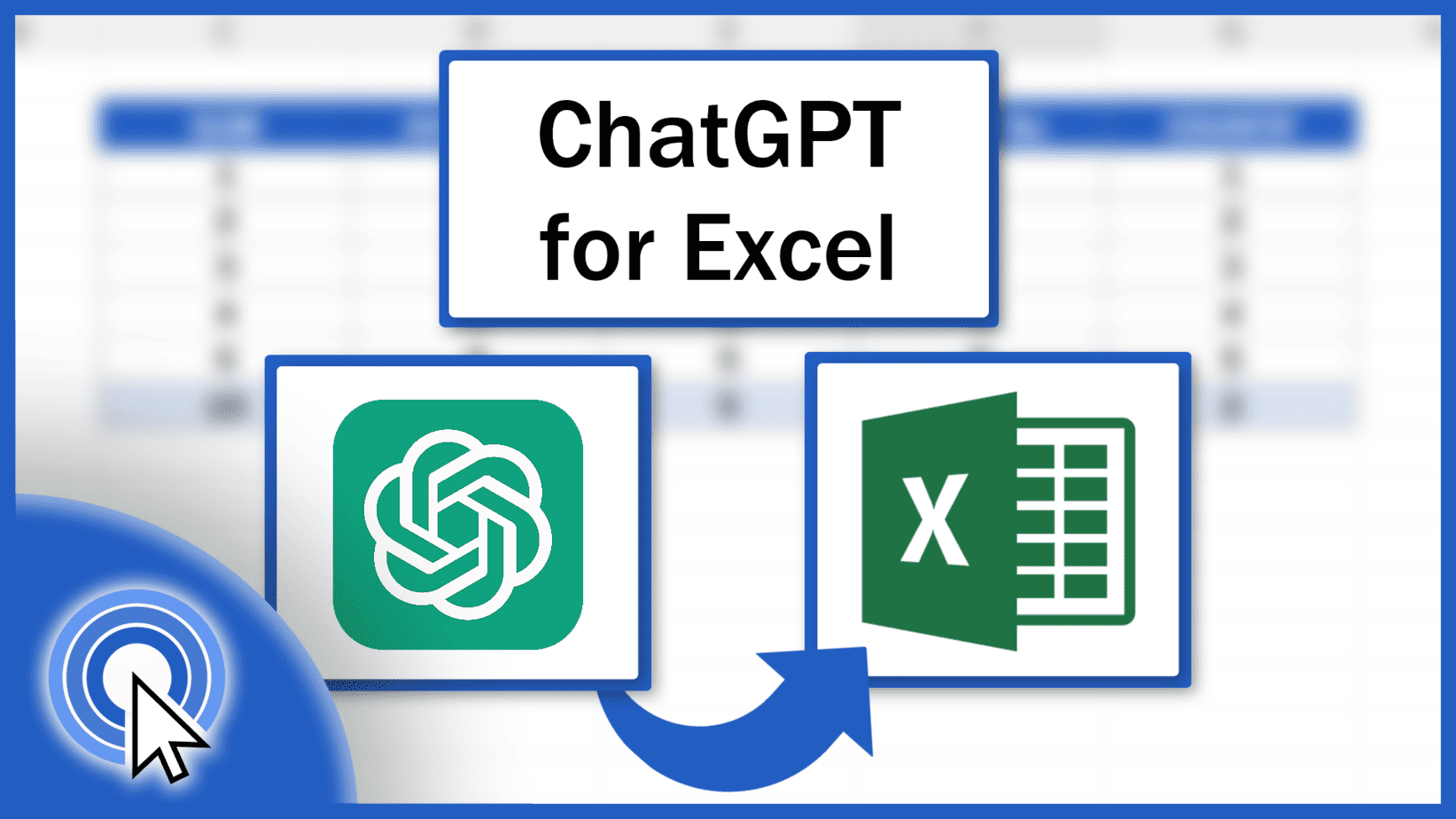## How to Integrate ChatGPT into Excel (Easy Integration)

In this tutorial, we’re going to have a look at how to integrate ChatGPT into Excel in a simple and easy way. Thanks to AI, you’ll be able to use Excel more effectively every day!## No Stress About Excel Formulas – Let AI Explain (Inside Excel)

This tutorial shows how we can use AI, specifically ChatGPT, to help us understand what exactly the Excel formula we just used does.## ChatGPT for Excel: How to Create Excel Formulas – Super Easy with AI

Today we’ll have a look at how to create Excel formulas in a super easy way using ChatGPT. Thanks to artificial intelligence, we can make use of Excel functions even more effectively.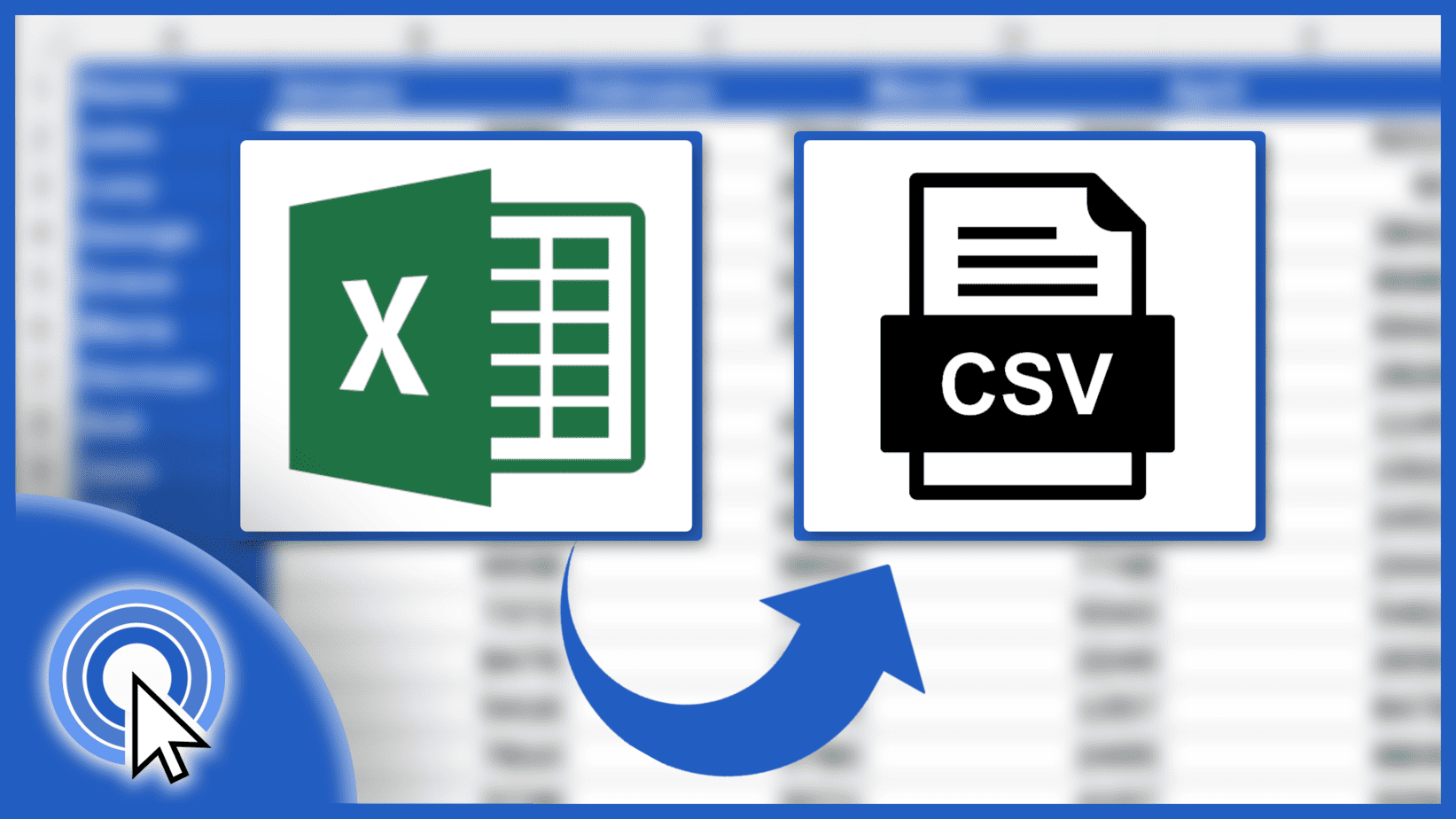## How to Convert Excel to CSV (Quick and Correct)

In this tutorial we’re going to have a look at how to convert an Excel spreadsheet to a CSV file in a quick and simple way.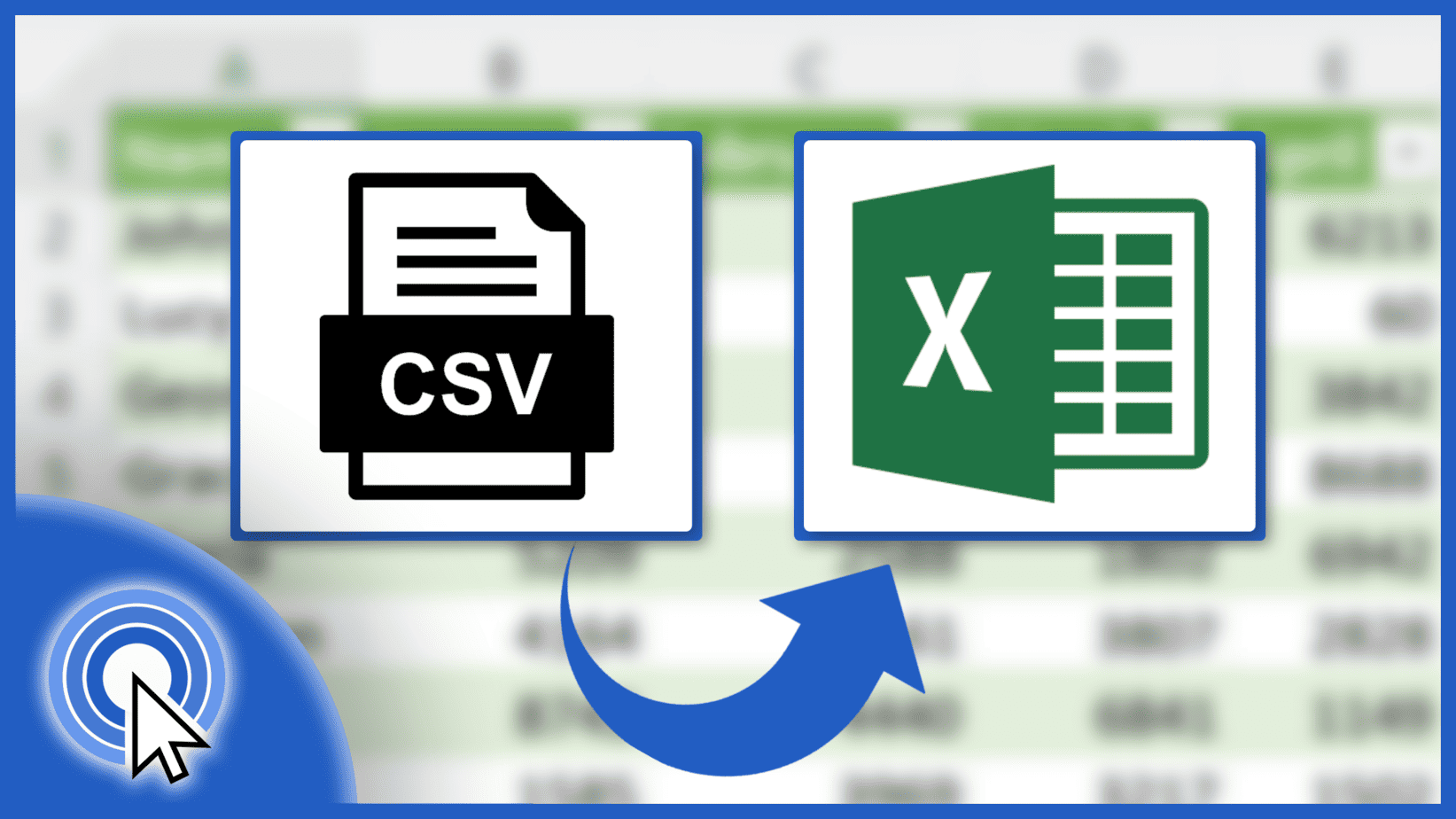## How to Convert CSV to Excel (Simple and Quick)

Today we’ll be talking about how to convert a CSV file to an Excel spreadsheet in a quick and simple way. Let’s have a look now!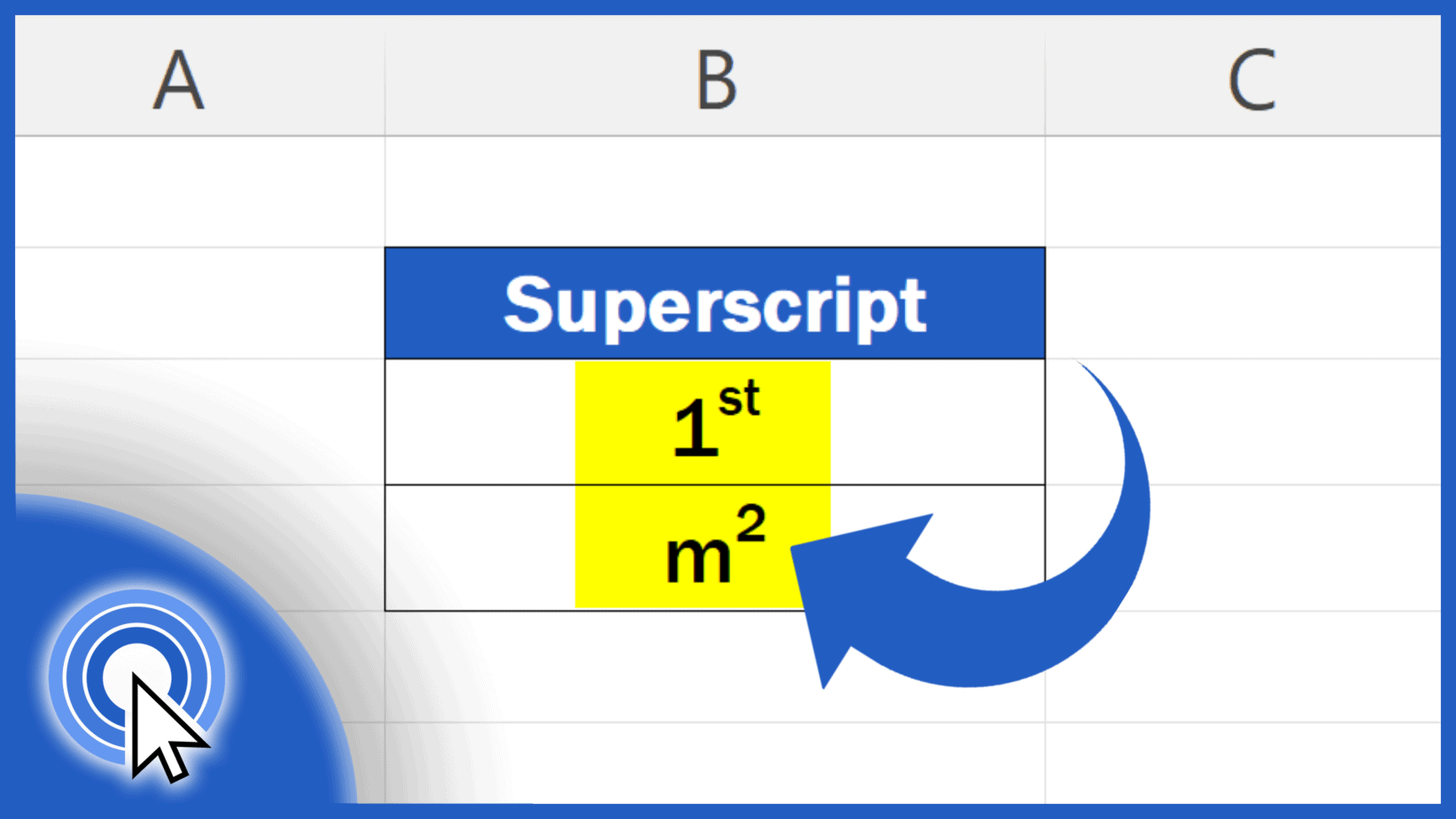## How to Add Superscript in Excel (the Simplest Way)